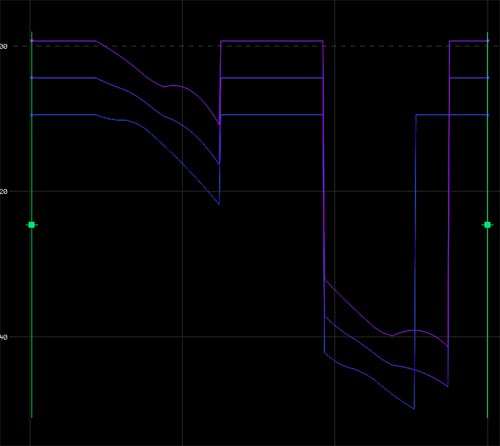## Recommended Posts

Hi, there.

I have a CHOP the snapshot of which is attached. It has periods of constant values mixed with periods of changing values. What I need to do is to "normalize" changing values so that they are equal to the first changing value in each period. Please, advise, because I'm out of ideas. Thank you very much,

Luka##### Share on other sites

How did you generate this channel in the first place? If it was synthesized in CHOPs, then there is a good chance you can level the periods of constant with the outgoing changing segment.

After the fact, it is a bit tough.

My initial idea is to force the flat regions to be zero then add back the last changing value to the constant segment. For this, you have to make two big assumptions:

- The areas of zero slope are just that

- The areas of changing value have no values that rest at 0

If that is the case, follow your channel with the following chops:

slope CHOP

> set at defaults. Isolates the areas of change

logic CHOP

> Convert Input set to "Off When Outside Bounds"

> Channel Pre OP set to "Toggle"

Then wire the output of your initial channel and the logic CHOP in to a math then multiply. Now you have all your flat regions at zero.

Now you have to do something like: if(\$V==0, `\$V-1`, \$V). VOPs or expression chop but I know that this will fail in the espression chop as is as backquotes don't behave as I thought (cause a red flag).

I have other ideas but no time right now.

##### Share on other sites

Hey, Old School, thanx! I tried it out and the expression didn't work for me either, but it gave me some food for thought. Will try to do it with VOPs.If youhave any other ideas, though, please let me know, I'll be very grateful.

Luka

##### Share on other sites

I have another question - let's say I have an object that is animated with 2 chops to make a circle. Is there some way to calculate the diameter of this circle?

Thanks a lot.

##### Share on other sites

That depends on how your circle is located in space. I assume, that the center is at the origin and the object moves in the x-y-plane. Then the two animation-curves satisfy the following condition:

x = r*sin(t)

y = r*cos(t)

Then you can solve this system of equations for the radius r. The result is:

r = x/(sin(arctan(x/y))

If your circle is offset from the center or doesn't lie in a coordinate-plane you can't find such a simple solution, since you have and underdetermined system (more unknowns than equations). An approach to that yould be to look at various positions in time on the circle, to get as much equations as you have unknowns. Then you are able to compute the radius again. But that's quite some math.

## Create an account

Register a new account